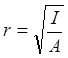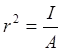# Geometric Properties of Areas

There are several geometric properties of the cross-section of a structural member which are important in terms of the member’s behavior under loads:

Area or Cross Sectional Area (A): This is the area of the section when cut perpendicular to the longitudinal (or x-) axis. It is easy to compute this property which also has an explicit physical meaning. In terms of the behavior of a structural member, A, represents the axial and shear stiffness.

Principal Axes and Centroid: The principal axes are the main axes of a member which are perpendicular and intersect each other at the center of area or “centroid”. One can think of the centroid as the center of gravity of an object if it is made of one material (homogeneous).

Moment of Inertia (I): Moment of inertia does not have an explicit physical meaning, i.e., one cannot observe it. However, it represents the bending stiffness of the structural member, i.e., how difficult it is to bend it. A higher value for a member’s moment of inertia translates to a stiffer member. Since there are two bending axes in a two-dimensional space(y and z), we have two values of moment of inertia defined about each axis. It is usually measured in units of (in4).

In addition to the moments of inertia about the two main axes, we have polar moment of inertia, J, which represents the stiffness of circular members such as solid shafts and hollow structural sections against torsion.

Torsion of a Solid Shaft

Torsion of a Hollow Structural Section

Torsion of a hollow structural section
For members with non-circular cross section the torsional stiffness is not related to the polar moment of inertia. For those shapes a “torsional constant” is usual.

Radius of Gyration (r): Similar to the moment of inertia, radius of gyration does not have an explicit physical meaning. The radius of gyration is defined as:or“r” represents the radius of gyration and has units of inches. Since there are two moments of inertia for bending about the y and z axes, we typically have two different radii of gyration, defined as:

Radius of gyration represents the resistance of columns against buckling. The larger the radius of gyration, the more resistance a column has against buckling as its slenderness ratio becomes smaller.